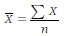# Calculations and Basic Logarithms

## Calculation of the Mean1

To determine the mean of a population or sample, all of the data are simply added together and divided by the number of pieces of data. For example, suppose that three tests of the resolving power of a film produced the following data: 59 lines/mm, 55 lines/mm, and 51 lines/mm. Using the formula(where the symbol Ʃ means “sum of”) the steps are:

1.Add up all of the X values: (59 + 55 + 51 = 165).

2.Divide the total by the number of X values: (n) (165 3 = 55.0).

Notice that the mean is carried to one more decimal place than the original data. Thus, the mean resolving power ...

Get Basic Photographic Materials and Processes, 3rd Edition now with the O’Reilly learning platform.

O’Reilly members experience books, live events, courses curated by job role, and more from O’Reilly and nearly 200 top publishers.